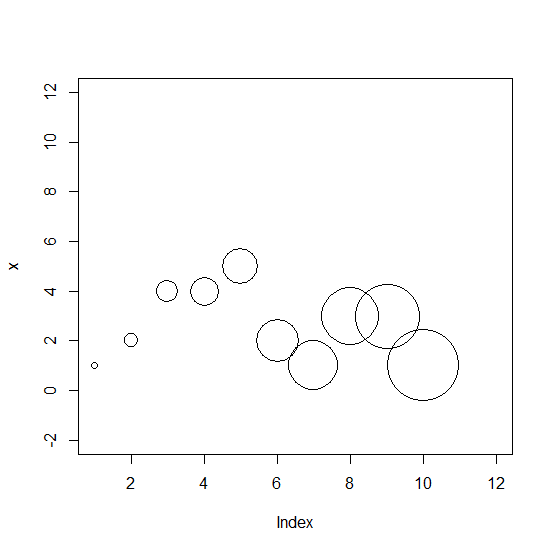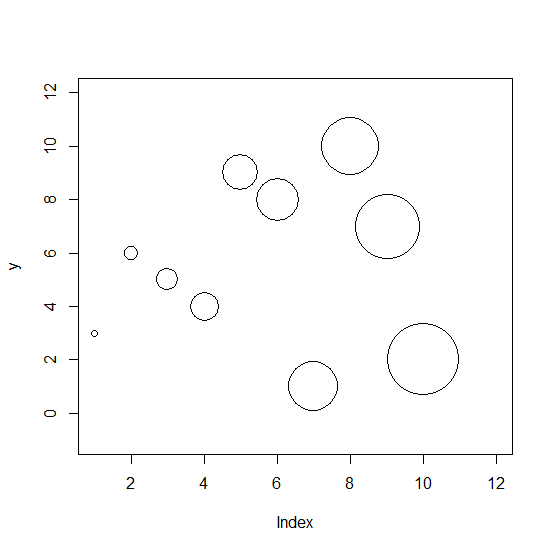# How to create a point chart with point size increment based on the position of the point in R?

The cex argument is used to increase or decrease the point size in a point chart created in base R. If we want to create a point chart with points of size in increment manner then we can pass a vector of the same size as the vector for which we want to create the point chart. For example, if we have a vector x that contains 10 elements then cex will be set as cex=1:10.

## Example1

Live Demo

> x<-rpois(10,2)
> plot(x,cex=1:10,xlim=c(1,12),ylim=c(-2,12))

## Output:## Example

Let’s have a look at another example:

Live Demo

> y<-sample(1:10,10)
> plot(y,cex=1:10,xlim=c(1,12),ylim=c(-1,12))

## Output: